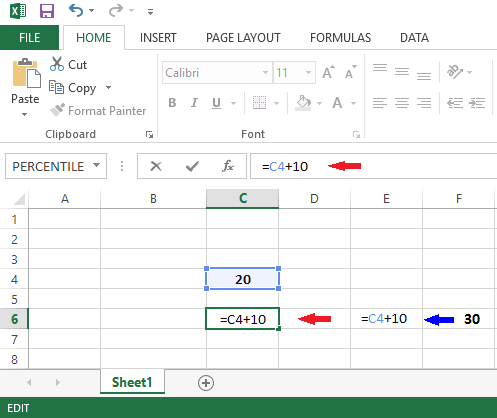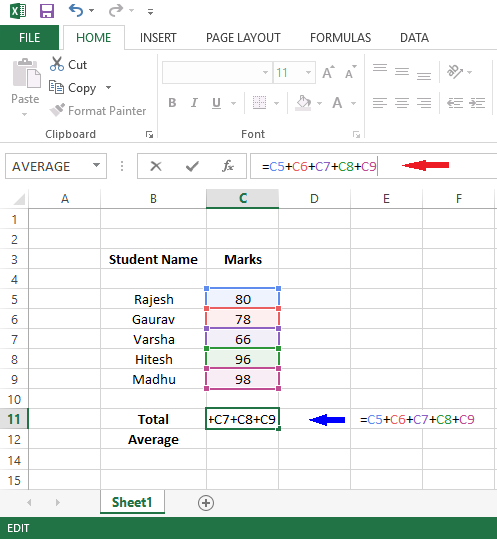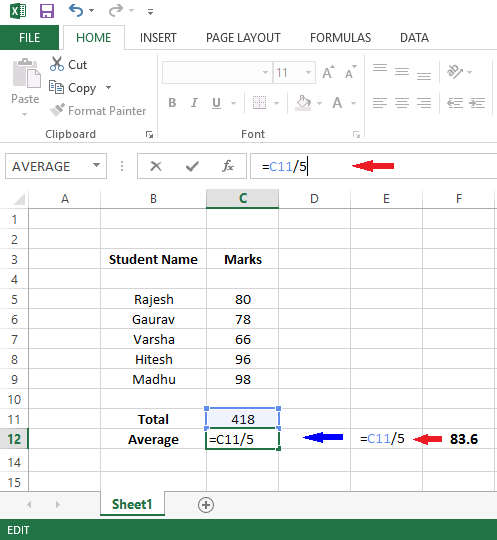# Create Formula in Excel

## How to Create Formula in Excel

Excel is frequently used in any corporate sector as well as in small office for data manipulation. Create won formula for calculation is one of the main feature of excel. Here we discuss about how to create formula in excel.

To Create formula in Excel first put = sign in any cell where you want to formula. Next put your formula according to your requirement.

In cell C4 enter the number 20, now in cell C6 type the formula: =C5+10 then press the Enter key. You will get the result in cell C6. Here formula says 10 is added to the value entered in the cell C4 as the value is 20 the result is 30. You can see in below image;In below image you can see we create formula for addition of students marks (=C5+C6+C7+C8+C9) and result will display in C11.In below image you can see we create formula for find average of students marks (=C11/5) and result will display in C12.More formulas can be created by typing = in the cell then selecting the appropriate values and typing correct mathematical symbol in between them.